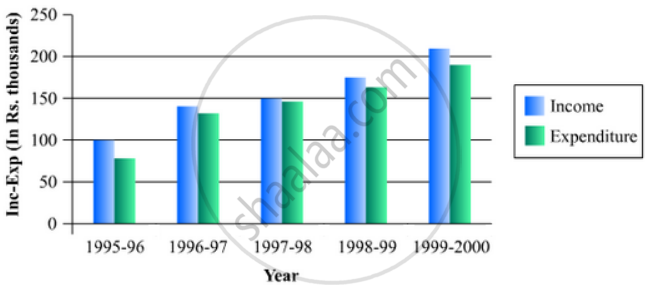# The Income and Expenditure for 5 Years of a Family is Given in the Following Data: Represent the Above Data by a Gar Graph. - Mathematics

The income and expenditure for 5 years of a family is given in the following data:

 Years 1995-96 1996-97 1997-98 1998-99 1999-2000 Income(Rs. inthousands) 100 140 150 170 210 Expenditure(Rs. in thousands) 80 130 145 160 190

Represent the above data by a gar graph.

#### Solution

To represent the given data by a vertical bar graph, we first draw horizontal and vertical axes. Let us consider that the horizontal and vertical axes represent the years and the income or expenditure in thousand rupees respectively. We have to draw 5 bars for each income and expenditure side by side of different lengths given in the table.

At first we mark 5 points for each income and expenditure in the horizontal axis at equal distances and erect rectangles of the same width at these points. The heights of the rectangles are proportional to the corresponding income or expenditures.

The vertical bar graph of the given data is following:Concept: Graphical Representation of Data
Is there an error in this question or solution?

#### APPEARS IN

RD Sharma Mathematics for Class 9
Chapter 23 Graphical Representation of Statistical Data
Exercise 23.2 | Q 13 | Page 27

Share